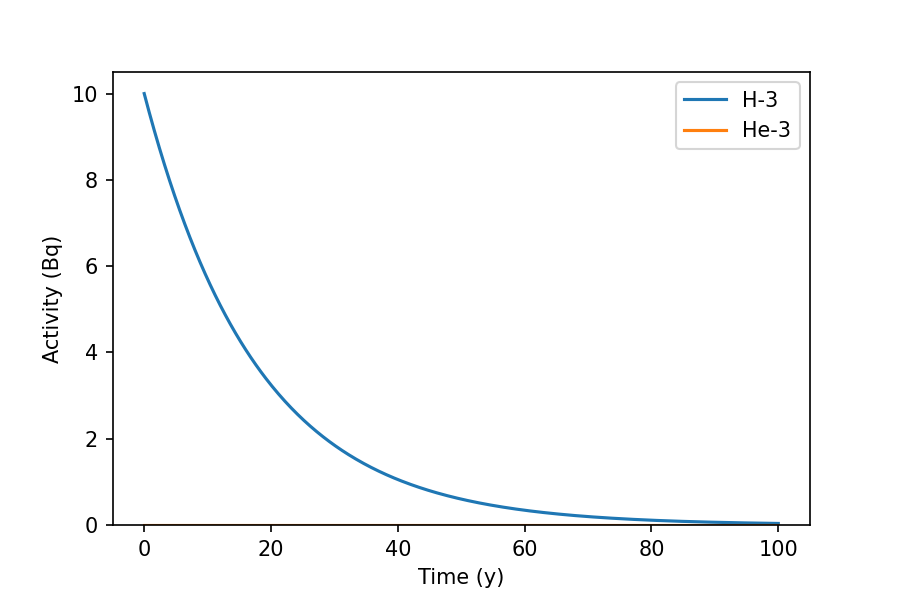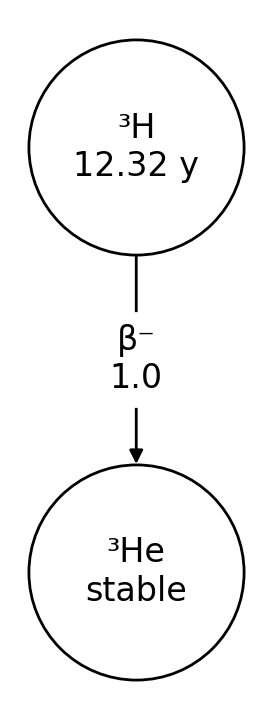# Overview

## Introduction

`radioactivedecay` is a Python package for radioactive decay calculations. It contains functions to define inventories of radionuclides, perform decay calculations, and output decay data for radionuclides and decay chains.

The original goal was to create a light-weight Python package for radioactive decay calculations, with full support for branching decays, multi-step decay chains, and metastable states. By default `radioactivedecay` uses decay data from ICRP Publication 107  and atomic mass data from the Atomic Mass Data Center (AME2020 and Nubase 2020 evaluations) ,  and . It solves the radioactive decay differential equations analytically using basic linear algebra operations .

In order to use `radioactivedecay`, you will need Python 3.6+ with the Matplotlib, NetworkX, NumPy, SciPy, Setuptools and SymPy packages installed. The code is platform independent and has been tested on Windows, MacOS and Linux systems.

## Quick start

Install `radioactivedecay` using `pip` by:

```\$ pip install radioactivedecay
```

or using `conda` by:

```\$ conda install -c conda-forge radioactivedecay
```

Either command will attempt to install the dependencies (Matplotlib, NetworkX, NumPy, SciPy, Setuptooks & SymPy) if they are not already present in the environment.

Import the `radioactivedecay` package and decay a simple inventory using:

```>>> import radioactivedecay as rd
>>> inv_t0 = rd.Inventory({'H-3': 10.0}, 'Bq')
>>> inv_t1 = inv_t0.decay(12.32, 'y')
>>> inv_t1.activities()
{'H-3': 5.0}
```

Here we created an inventory of 10.0 Bq of tritium (3H) and decayed it for 12.32 years. The activity reduced by a factor of two, i.e. to 5.0 Bq, as 12.32 years is the half-life of tritium.

Additional options for inputs and outputs include masses, moles and numbers of atoms. Use the `units` argument to the `Inventory()` constructor, and the `masses()`, `moles()`, `numbers()`, `mass_fractions()`, and `mole_fractions()` methods to obtain results.

```>>> inv_mass_t0 = rd.Inventory({'H-3': 3.2}, 'g')
>>> inv_mass_t1 = inv_mass_t0.decay(12.32, 'y')
>>> inv_mass_t1.masses('g')
{'H-3': 1.6000000000000003, 'He-3': 1.5999894116584246}

>>> inv_mol_t0 = rd.Inventory({'C-14': 1.0}, 'mol')
>>> inv_mol_t1 = inv_mol_t0.decay(3000.0, 'y')
>>> inv_mol_t1.moles('mol')
{'C-14': 0.6943255713073281, 'N-14': 0.3056744286926719}
>>> inv_mol_t1.numbers()
{'C-14': 4.181326323680147e+23, 'N-14': 1.840814436319853e+23}
>>> inv_mol_t1.mole_fractions()
{'C-14': 0.6943255713073281, 'N-14': 0.3056744286926719}
```

Use the `plot()` method to show the decay of the inventory over time:

```>>> inv_t0.plot(100, 'y')
```The graph shows the decay of H-3 over a 100 year period.

Use the `Nuclide` class to fetch decay and atomic data about a particular nuclide and to draw diagrams of its decay chain:

```>>> nuc = rd.Nuclide('H-3')
'12.32 y'
>>> nuc.progeny()
['He-3']
>>> nuc.decay_modes()
['β-']
>>> nuc.branching_fractions()
[1.0]
>>> nuc.Z  # proton number
1
>>> nuc.A  # nucleon number
3
>>> nuc.atomic_mass  # atomic mass in g/mol
3.01604928132
>>> nuc.plot()
```## How it works

`radioactivedecay` calculates an analytical solution to the decay chain differential equations using matrix and vector multiplications. It implements the method described in ref. . See the theory docpage for more details. It calls NumPy  and SciPy  for the matrix operations. There is also a high numerical precision decay calculation mode based on SymPy  routines.

The datasets repo on GitHub contains a Jupyter Notebook for creating the ICRP-107 decay dataset. Notebooks with cross-checks of decay calculation results against PyNE  and Radiological Toolbox  are held in the comparisons repo.

## Limitations

At present `radioactivedecay` has the following limitations:

• It does not model neutronics, so cannot calculate radioactivity produced from neutron-nuclear reactions inducing radioactivity or fission.

• It cannot model temporal sources of external radioactivity input or removal from an inventory over time.

• Care is needed when decaying backwards in time, i.e. supplying a negative argument to the `Inventory.decay()` method, as this can result in numerical instabilities and nonsensical results. The high precision `InventoryHP.decay()` is more robust to these instabilities, but not immune from them.

There are also some limitations associated with the ICRP-107 decay dataset:

• ICRP-107 does not contain data on branching fractions for radionuclides produced from spontaneous fission decays. Thus the `decay()` methods do not calculate activities for spontaneous fission progeny.

• Decay data is quoted in ICRP-107 with up to 5 significant figures of precision. The results of decay calculations will therefore in theory never be more precise than this level of precision.

• Uncertainties are not quoted for the radioactive decay data in ICRP-107. Uncertainties will vary substantially between radionuclides. They will depend on how well each radionuclide has been researched in the past. In many cases these uncertainties will be more significant for the results of decay calculations than the previous point about the quoted precision of the ICRP-107 decay data.

• There are a few instances where minor decay pathways were not included in ICRP-107, e.g. the decay pathways for At-219-> Rn-219 (β ~3%), Es-250 -> Bk-246 (α ~1.5%), and U-228 -> Pa-228 (ε ~2.5%). For more details see refs.  and  on the creation of the ICRP-107 dataset.

`radioactivedecay` is open source software released under the MIT licence.

The default decay data used by `radioactivedecay` is ICRP-107 , which is Copyright © A. Endo and K.F. Eckerman (2008). See LICENSE.ICRP-07 for more details.

The default atomic mass data is based on the Atomic Mass Data Center (AMDC) AME2020 ,  and Nubase2020  evaluations. See LICENSE.AMDC for more details.

## Citation

If you find this package useful for your research, please consider citing the paper on `radioactivedecay` published in the Journal of Open Source Software:

A. Malins & T. Lemoine, radioactivedecay: A Python package for radioactive decay calculations. Journal of Open Source Software, 7 (71), 3318 (2022). DOI: 10.21105/joss.03318.

## Contributors

List of contributors to `radioactivedecay`:

## Contributing

Users are welcome to fix bugs, add new features or make feature requests. Please read the contributor guidelines and open a pull request or issue on the GitHub repository.

## Queries and suggestions

If you have any questions or suggestions, please post on the Discussions page on GitHub.

## Acknowledgements

Special thanks to:

for suggestions, support and assistance to this project.

Thanks also to:

for their work on open source radioactive decay calculation software.